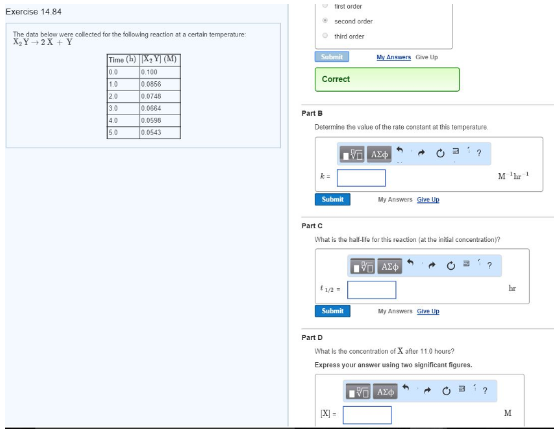# Problem: The data below were collected for the following reaction at a certain temperature: X2Y → 2X + Y Determine the value of the rate constant at this temperature. What is the half-life for this reaction (at the initial concentration)? What is the concentration of X after 11.0 hours?Express your answer using two significant figures.

###### FREE Expert Solution
85% (333 ratings)###### Problem Details

The data below were collected for the following reaction at a certain temperature:

X2Y → 2X + Y

Determine the value of the rate constant at this temperature.

What is the half-life for this reaction (at the initial concentration)?

What is the concentration of X after 11.0 hours?